## Day 1, Problem 3 - Wisdom Beads Game

The wisdom beads game set consists of a triangular board and 12 game pieces of varying shapes. The board is depicted in figure 1, as follows: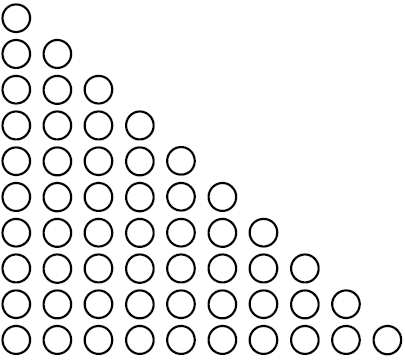Figure 1

The 12 game pieces fall into 3 major categories, depending on the number of beads they consist of.

For the 1st major category, there are 3 beads, but only one type of shape:

The symbol is A, the shape is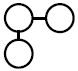For the 2nd major category, there are 4 beads and 3 types of shapes:

The symbol is B, the shape is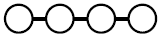The symbol is C, the shape is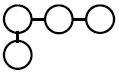The symbol is D, the shape is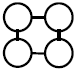For the 3rd major category, there are 5 beads and 8 types of shapes:

The symbol is E, the shape is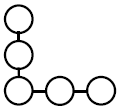The symbol is F, the shape is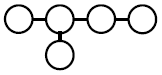The symbol is G, the shape is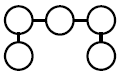The symbol is H, the shape is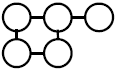The symbol is I, the shape is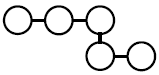The symbol is J, the shape is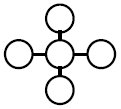The symbol is K, the shape is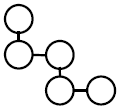The symbol is L, the shape is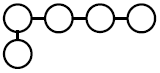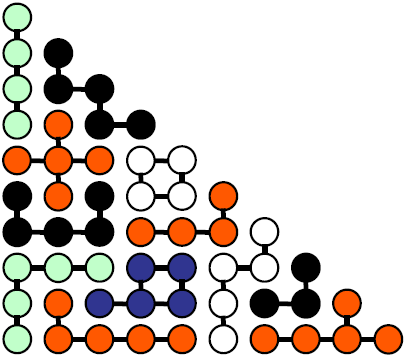Figure 2

Figure 2 depicts one method of piecing together the game board. The depiction in figure 2 can be simplified to figure 3, so the board can be expressed as a two-dimensional array of characters.

 ``` B B K B K K B J K K J J J D D G J G D D C G G G C C C I E E E H H I I A E L H H H I A A F E L L L L I F F F F``` Figure 3

A may be placed at any location on the board, provided that there is room to place it, and that the dimensions are appropriate. Rotations (0°, 90°, 180°, and 270°) and flips (horizontal and vertical) are allowed for placing any of the pieces.

Given the initial setup of a game board, determine a valid method for placing all of the remaining pieces onto the board.

### Input Format

The input contains a total of 10 lines, describing the initial game board. Line i contains i characters. If the j-th character of line i is a letter from '`A`' to '`L`', then that means row i column j has already been occupied by the game piece of the specified letter. If the j-th character of line i is the character '`.`', then that means row i column j is currently empty.

### Output Format

If it's possible to find a solution, print 10 lines to the output - the game board after all 12 pieces have been placed. The i-th line must contain i characters, where the j-th character of the i-th line is the symbol of the piece that occupies the board at that location.
If there's no solution, output a single line with the string "`No solution`" (do not output quotation marks).
The data guarantees that there will be at most one solution for each test case.

```.
..
...
....
.....
.....C
...CCC.
EEEHH...
E.HHH....
E.........
```

### Sample Output

```B
BK
BKK
BJKK
JJJDD
GJGDDC
GGGCCCI
EEEHHIIA
ELHHHIAAF
ELLLLIFFFF
```

Point Value: 40 (partial)
Time Limit: 2.00s
Memory Limit: 256M